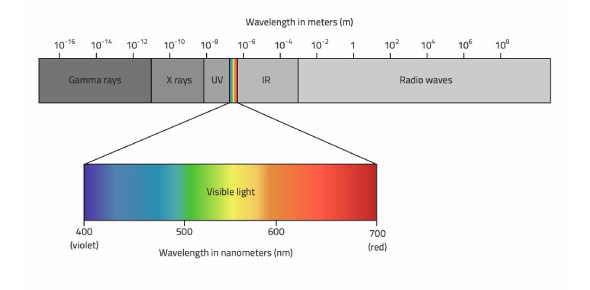# Ems Recall

23 Questions | Total Attempts: 1250Settings.

• 1.
A group of wavelengths (or) frequencies is known as:
• A.

Group of colours

• B.

Spectrum

• C.

Colour band

• D.

None

• 2.
• A.

Mechanical

• B.

Longitudinal

• C.

Transverse

• D.

Stationary

• 3.
The longest wavelength of the spectrum.
• A.

• B.

UV Waves

• C.

Visible Light

• 4.
The shortest wavelength of the spectrum.
• A.

Microwaves

• B.

Infrared Waves

• C.

Gamma Rays

• 5.
This type of electromagnetic radiation is the most dangerous to humans:
• A.

X-rays

• B.

Gamma rays

• C.

Microwaves

• D.

Infrared waves

• 6.
Electromagnetic waves are transverse waves that transfer
• A.

Sound energy

• B.

Photons

• C.

Electrical energy

• D.

Magnets

• 7.
Which of the following is not an example of electromagnetic radiation?
• A.

Dangerous Gamma Rays

• B.

Photons of energy traveling through space

• C.

The sonic boom of a jet

• D.

Wi-fi signals

• 8.
The distance between A and F is the _____________.
• A.

Frequency

• B.

Period

• C.

Amplitude

• D.

Wavelength

• 9.
The amount of energy carried by an electromagnetic wave increases with frequency.  Which of the following groups of waves is listed in order of increasing energy?
• A.

• B.

Infrared waves, visible light, radio waves

• C.

• 10.
Which of the following emits infrared radiation(s)?
• A.

Hot iron

• B.

Ice

• C.

Freezing mixture

• D.

Fire

• E.

Sunlight

• 11.
ROY G BIV is what part of the EMS?
• A.

Gamma rays

• B.

• C.

Visible Light

• 12.
Produced by dying stars, decaying nuclei, and used in killing cancer cells.
• A.

Visible Light

• B.

Infrared Waves

• C.

Gamma Rays

• 13.
How much of the electromagnetic spectrum is visible to just the human eye?
• A.

All of it (radio, microwaves, infrared, visible light, UV, X-rays, gamma rays)

• B.

None of it (no rays)

• C.

Most of it (only radio, infrared, x-rays, gamma rays)

• D.

Only a small part (visible light)

• 14.
This color has the longest wavelength of visible light:
• A.

Red

• B.

Orange

• C.

Yellow

• D.

Green

• E.

Blue

• F.

Indigo

• G.

Violet

• 15.
What is the Electromagnetic Spectrum?
• A.

The range of EM waves according to their frequencies

• B.

The vibration of electrically charged particles that surround the electric field

• C.

Range of chemical formulas based on nomenclature

• 16.
Visible light is light that humans can see. What is the range of colors in order from short to long wavelength?
• A.

Violet, indigo, green, red, orange

• B.

Red, Orange, Yellow, Green, Blue, Indigo, and Violet

• C.

Violet, indigo, blue, green, yellow, orange, red

• 17.
Light travels in...
• A.

A limo

• B.

Curved lines. If it didn't, we wouldn't be able to see.

• C.

Straight lines

• D.

By bus. It's the easiest, cheapest and most economical way.

• E.

The day time

• 18.
The distance between D and I is the _____________.
• A.

Frequency

• B.

Trough

• C.

Amplitude

• D.

Wavelength

• 19.
A and F are what part of the wave?
• A.

Crests

• B.

Troughs

• C.

Amplitudes

• D.

Wavelength

• 20.
D and I are what part of the wave?
• A.

Crests

• B.

Troughs

• C.

Amplitudes

• D.

Wavelength

• 21.
Which electromagnetic waves are used to kill bacteria?
• A.

Gamma

• B.

X-rays

• C.

UV

• D.

Infrared

• 22.
Which waves on the electromagnetic spectrum are considered not dangerous?
• A.

• B.

Gamma waves

• C.

Infrared waves

• D.

Ultraviolet waves

• E.

Visible light

• 23.
In the Electromagnetic Spectrum, the waves with higher frequencies have __________ energy.
• A.

Higher

• B.

Lower

• C.

No change in in energy they all travel at the speed of light in a vacuum (can't trick me)

Related TopicsBack to top